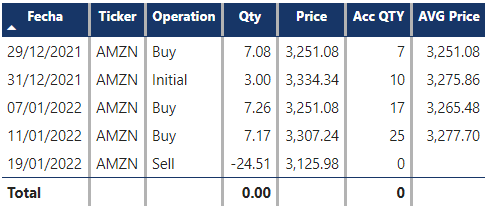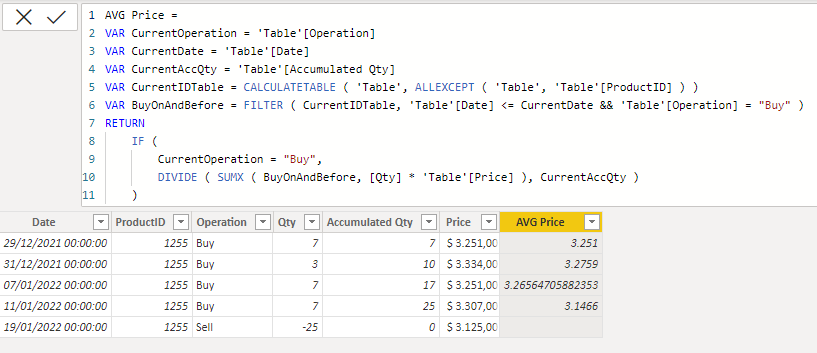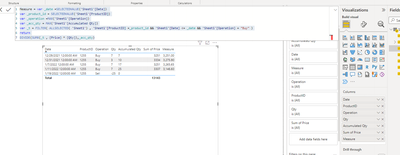cancel
Showing results for
Did you mean:Frequent Visitor

## Average Purchase Price in every instant

Hello All!

I'm working with a fact table and need to get the average purchase price for every instant.

What I need to do is multiply the price of every purchase for the quantity and divide that result in the accumulated quantity considering the same for the previous purchases, as the excel example below:

 Date ProductID Operation Qty Accumulated Qty Price AVG Price Example 29/12/2021 00:00 1255 Buy 7 7 \$ 3.251,00 \$ 3.251,00 7*3251/7 31/12/2021 00:00 1255 Buy 3 10 \$ 3.334,00 \$ 3.275,90 3*3334/10 + 7*3251/10 7/1/2022 00:00 1255 Buy 7 17 \$ 3.251,00 \$ 3.265,65 7*3251/17 + 3*3334/17 + 7*3251/17 11/1/2022 00:00 1255 Buy 7 25 \$ 3.307,00 \$ 3.146,60 7*3307/25 + 7*3251/25 + 3*3334/25 + 7*3251/25 19/1/2022 00:00 1255 Sell -25 0 \$ 3.125,00

I wasn't able to replicate this in PBI, can anyone help me with this?

1 ACCEPTED SOLUTIONSuper User

Hi @AndresOHV
Please refer to attched sample file with the proposed solution. Please let me know if this is as per your requirement or something else is required.``````Acc QTY =
CALCULATE (
[Qty],
FILTER (
ALL ( 'Calendar' ),
'Calendar'[Fecha] <= MAX ( 'Calendar'[Fecha] )
),
ALL ( FactExample[Operation] ),
ALL ( FactExample[Price] )
)``````
``````AVG Price =
VAR CurrentDate = MAX ( 'Calendar'[Fecha] )
VAR CurrentOperation = SELECTEDVALUE ( FactExample[Operation] )
VAR AccumulatedQty = [Acc QTY]
VAR T1 =
CALCULATETABLE (
SUMMARIZE (
FactExample,
Ticker[Ticker],
'Calendar'[Fecha],
FactExample[Price],
FactExample[Operation]
),
REMOVEFILTERS ( ),
VALUES ( FactExample[Ticker] ),
FactExample[Operation] <> "Sell" ,
'Calendar'[Fecha] <= CurrentDate
)
VAR T2 =
T1,
"@Qty", [Qty]
)
RETURN
IF (
CurrentOperation <> "Sell",
DIVIDE (
SUMX ( T2, [@Qty] * [Price] ),
AccumulatedQty
)
)``````
10 REPLIES 10Super User

Hi @AndresOHV
Please refer to attached sample file with the solution``````AVG Price =
VAR CurrentOperation = 'Table'[Operation]
VAR CurrentDate = 'Table'[Date]
VAR CurrentAccQty = 'Table'[Accumulated Qty]
VAR CurrentIDTable = CALCULATETABLE ( 'Table', ALLEXCEPT ( 'Table', 'Table'[ProductID] ) )
VAR BuyOnAndBefore = FILTER ( CurrentIDTable, 'Table'[Date] <= CurrentDate && 'Table'[Operation] = "Buy" )
RETURN
IF (
DIVIDE ( SUMX ( BuyOnAndBefore, [Qty] * 'Table'[Price] ), CurrentAccQty )
)``````Frequent Visitor

I'm trying to replicate this buy I'm having a "circular dependency" when creating the column.

The productId and the date come from 2 different dimension tables. Wouldn't be better to create a measure instead of a column?Super User

Hi @AndresOHV
Still not very clear, therefore, I made some assumptions again.``````AVG Price =
VAR CurrentDate = MAX ( 'Date'[Date] )
VAR CurrentOperation = SELECTEDVALUE ( Sales[Operation] )
VAR AccumulatedQty = [Accumulated Qty]
VAR T1 =
CALCULATETABLE (
SUMMARIZE (
Sales,
Sales[ProductID],
Sales[Price],
Sales[Operation],
'Date'[Date]
),
REMOVEFILTERS ( ),
VALUES ( 'Sales'[ProductID] ),
'Date'[Date] <= CurrentDate
)
VAR T2 =
T1,
"@Qty", [Qty]
)
RETURN
IF (
DIVIDE (
SUMX ( T2, [@Qty] * [Price] ),
AccumulatedQty
)
)``````Community Support

Hi , @AndresOHV

For measure , you can use this dax :

``````Measure = var _date =SELECTEDVALUE('Sheet1'[Date])
var _product_id = SELECTEDVALUE('Sheet1'[ProductID])
var _operation =MAX('Sheet1'[Operation])
var _acc_qty = MAX('Sheet1'[Accumulated Qty])
var _t = FILTER( ALLSELECTED( 'Sheet1') , 'Sheet1'[ProductID] =_product_id && 'Sheet1'[Date] <= _date && 'Sheet1'[Operation] = "Buy" )
return
DIVIDE(SUMX(_t , [Price] * [Qty]),_acc_qty)``````

The result is as follows:Best Regards,

Aniya Zhang

If this post helps, then please consider Accept it as the solution to help the other members find it more quicklyFrequent Visitor

I'm trying to use this but I get a different result. I think it's because you're using just one table and in the real model I have the date and product id in different tabels so I'm not sure how to make it work.Community Support

Hi , @AndresOHV

You are right , the measure need to write by the table relationship. So do you want to share the sample .pbix file to me so that we can help you better.

Best Regards,

Aniya Zhang

If this post helps, then please consider Accept it as the solution to help the other members find it more quicklyFrequent Visitor

Hi @v-yueyunzh-msft ! Thank you for your help.

I'm copying a link to the pbi file:

https://1drv.ms/u/s!AvSFZ2hooRIDgdJPNOk7duNVPTrnbQ?e=STE3XrSuper User

Hi @AndresOHV
Please refer to attched sample file with the proposed solution. Please let me know if this is as per your requirement or something else is required.``````Acc QTY =
CALCULATE (
[Qty],
FILTER (
ALL ( 'Calendar' ),
'Calendar'[Fecha] <= MAX ( 'Calendar'[Fecha] )
),
ALL ( FactExample[Operation] ),
ALL ( FactExample[Price] )
)``````
``````AVG Price =
VAR CurrentDate = MAX ( 'Calendar'[Fecha] )
VAR CurrentOperation = SELECTEDVALUE ( FactExample[Operation] )
VAR AccumulatedQty = [Acc QTY]
VAR T1 =
CALCULATETABLE (
SUMMARIZE (
FactExample,
Ticker[Ticker],
'Calendar'[Fecha],
FactExample[Price],
FactExample[Operation]
),
REMOVEFILTERS ( ),
VALUES ( FactExample[Ticker] ),
FactExample[Operation] <> "Sell" ,
'Calendar'[Fecha] <= CurrentDate
)
VAR T2 =
T1,
"@Qty", [Qty]
)
RETURN
IF (
CurrentOperation <> "Sell",
DIVIDE (
SUMX ( T2, [@Qty] * [Price] ),
AccumulatedQty
)
)``````Frequent Visitor

This worked @tamerj1 ! Thank you for your help.

Do you know how can I complete this measure so in the case of a Sell Operation rest the selling price to the calculated average?Super User

OK. This is rather simple and can be easily done in Power Query. But showing only one "Sell" entry and with 0 at that... does not help. You have to provide an example that's REPRESENTATIVE.